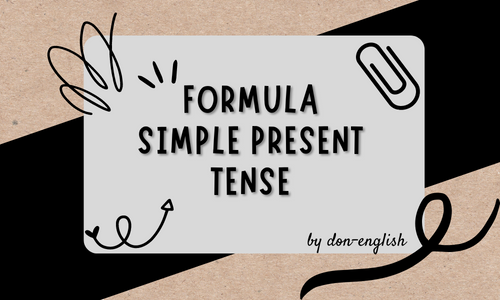Formula Simple Present Tense and Example in English - don-english
Lompat ke konten Lompat ke sidebar Lompat ke footer

# Formula Simple Present Tense and Example in English

The simple present tense formula is the basis for forming sentences using the simple present. There are many kinds of sentences that can be constructed using only a few formulas or rules. Indeed, that is the use of the formula or formula.

In this article, we will discuss thoroughly the verbal and nominal simple present tense formulas along with complete examples.Formula Simple Present Tense and Example in English

## The Formula of Simple Present Tense and Examples

Table of content:
• Verbal sentence formula
• Nominal sentence formula
• Positive sentence formula
• Negative sentence formula
• Interrogative sentence formula
• Passive voice simple present
• Closing

### Formula Simple Present Tense Verbal

Verbal sentences are sentences that use a predicate in the form of a verb. In this case, the predicate used is in the form of V1 either with s/es/ies or not.

If the subject used is a plural subject, it is enough to use V1 without the addition of s. However, if the subject used is a single subject, then use V1 with the addition of s/es/ies.

The following is a formula for positive, negative, and interrogative sentences for verbal sentences.

#### 1. Positive sentence formula

Positive sentences are sentences in the form of statements that use the subject + predicate + object/complement pattern.
• Single subject + V1(s/es/ies/etc) + object/complement
• Plural subject + V1 + object/complement
Example of sentences:
• I love you.
• She always goes there.
• Every Monday, the students go to school at 6.
• They usually read a book in the library.
• My mother gives me money every morning.

#### 2. Negative sentence formula

Negative sentences use do not/don't before the verb if the subject is plural. Meanwhile, if you use a single subject, you use does not/doesn't. Both still use V1 without any affixes, either singular or plural.
• Single S + do not/don't + V1 + object/complement
• S plural + does not/doesn't + V1 + object/complement
Examples:
• I don't know where you live.
• The teacher doesn't want our money.
• Every boy here doesn't go to the party.

#### 3. Question sentence formula

Interrogative sentences consist of two types, namely interrogative sentences with yes/no answers or commonly known as yes/no questions and questions with question words or question words. Both use the auxiliary do/does and the verb used is V1 without any affixes.

QW can be 5W1H (what, where, when, who, why, how) or other question words.
• Do + S plural + V1 + object/complement + ?
• Does + single S + V1 + object/complement + ?
• QW + do + S plural + V1 + object/complement + ?
• QW + does + S singular + V1 + object/complement + ?
Examples:
• Do you know my name?
• Does your brother study here?
• Where do you live?
• With whom does she usually go to school?

### Formula Simple Present Tense Nominal

Nominal sentences are sentences whose predicates use to be. In this tense, the to be used is to be present, namely is, am, and are.

"Is" is used for singular subjects, "am" is used for my subjects (I), and "are" is used for plural subjects. After to be, it will be followed by an adjective, a noun, or an adverb.

The following is a simple present tense nominal formula,

#### 1. Positive sentence formula

Nominal positive sentences use to be present followed by adverbs or nouns or adjectives.
Examples:
• I am a student.
• She is always at school at 6.
• We are usually together at the party.
• The dog is under the tree.

#### 2. Negative sentence formula

As with positive sentences, negative sentences only add the word not to is not, am no, are not or shortened to isn't, aren't, and am not there is no abbreviation.
Examples:
• She isn't my sister.
• We are not there when you are there.
• I am not the best player here.
• Somebody is in the class.

#### 3. Interrogative sentence formula

Similar to verbal sentences, nominal sentences have two forms, namely yes/no questions and questions with question words.

For yes/no questions, use the prefix to be present followed by the subject. Meanwhile, for questions with question words, use the question word prefix and then follow to be present.
Examples:
• Are you always here?
• Is she usually to school by bicycle?